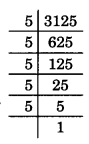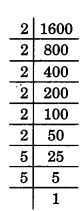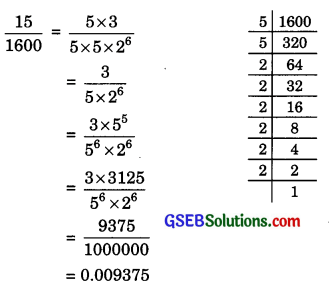# GSEB Solutions Class 10 Maths Chapter 1 Real Numbers Ex 1.4

Gujarat Board GSEB Solutions Class 10 Maths Chapter 1 Real Numbers Ex 1.4 Textbook Questions and Answers.

## Gujarat Board Textbook Solutions Class 10 Maths Chapter 1 Real Numbers Ex 1.4

Question 1.
Without actually performing the long division, state whether the following rational numbers will have a terminating decimal expansion or a non-terminating repeating decimal expansion.

1. $$\frac{13}{3125}$$
2. $$\frac{17}{8}$$
3. $$\frac{64}{455}$$
4. $$\frac{15}{1600}$$
5. $$\frac{29}{343}$$
6. $$\frac{23}{2^{3} \times 5^{2}}$$
7. $$\frac{129}{2^{2} \times 5^{7} \times 7^{5}}$$
8. $$\frac{6}{15}$$
9. $$\frac{35}{50}$$
10. $$\frac{77}{210}$$

Solution:
1. $$\frac{13}{3125}=\frac{13}{5^{5}}=\frac{13 \times 2^{5}}{5^{5} \times 2^{5}}$$
The denominator is of the form 2n × 5m.
Hence the decimal expansion of $$\frac{13}{3125}$$ is terminating.2. We can write $$\frac{17}{8}=\frac{17 \times 5^{3}}{2^{3} \times 5^{3}}=\frac{2125}{10^{3}}$$
the denominator is of the form 2n × 5m
Hence the decimal expansion $$\frac{17}{8}$$ terminating.

3. $$\frac{64}{455}$$We can write it $$\frac{64}{5^{1} \times 7^{1} \times 13^{1}}$$
Since the denominator is not in the form of 2n × 5m and it also contains 7 and 13 as factors. Its decimal expansion will be non-terminating repeating.

4. $$\frac{15}{1600}$$ = $$\frac{15}{2^{6} \times 5^{2}}$$
Denominator is of the form 2n × 5mHence the decimal expansion of $$\frac{15}{1600}$$ is terminating.

5. $$\frac{29}{343}$$ = $$\frac{29}{7^{3}}$$
Since the denominator is not in the form 2n × 5m and it also has 7 as factor then the decimal expansion of $$\frac{29}{343}$$ is non-terminating repeating.

6. $$\frac{23}{2^{3} \times 5^{2}}$$
The denominator is of the form 2n × 5m then decimal expansion is terminating.

7. $$\frac{129}{2^{2} \times 5^{7} \times 7^{5}}$$
Since in denominator 7 is a factor. Hence the decimal expansion of $$\frac{129}{2^{2} \times 5^{7} \times 7^{5}}$$ is non-terminating.8. $$\frac{6}{15}$$ = $$\frac{2 \times 3}{5 \times 3}=\frac{2}{5} \times \frac{2^{1}}{2^{1}}$$
Here denominator is in the form of 2n × 5m hence decimal expansion of $$\frac{6}{15}$$ is terminating.

9. $$\frac{35}{50}$$ = $$\frac{5 \times 7}{5 \times 5 \times 2}=\frac{7}{5^{2} \times 2^{1}}$$
Here denominator is in the form of 2n × 5m hence decimal expansion of $$\frac{35}{50}$$ is terminating.

10. $$\frac{77}{210}$$ = $$\frac{11 \times 7}{3 \times 7 \times 2 \times 5}=\frac{11}{3 \times 2 \times 5}$$
Since the denominator is not in the form of 2n × 5m and it also has 3 as a factor.
Then the decimal expansion of $$\frac{77}{210}$$ is non-terminating.

Question 2.
Write down decimal expansions of the rational numbers in question 1 above which have terminating decimal expansions.
Solution:
(i) $$\frac{13}{3125}$$ = $$\frac{13 \times 2^{5}}{5^{5} \times 2^{5}}$$
= $$\frac{416}{100000}$$(ii)(iii)(iv) $$\frac{23}{2^{3} \times 5^{2}}$$ = $$\frac{23 \times 5}{2^{3} \times 5^{3}}$$
$$\frac{115}{1000}$$ = 0.115(v) $$\frac{6}{15}$$ = $$\frac{2 \times 3}{5 \times 3}$$(vi) $$\frac{35}{60}$$ = $$\frac{5 \times 7}{5 \times 5 \times 2}$$
$$=\frac{7}{5 \times 2}=\frac{7}{10}=0.7$$

Question 3.
The following real numbers have decimal expansions as given below. In each case decide whether they are rational or not. If they are rational and of the form $$\frac{p}{q}$$ , what can you say about the prime factor of q?

1. 43.123456789
2. 0.120 1200 12000 120000 ……..
3. 43.1$$\overline { 23456789 }$$

Solution:
1. 43.123456789 = $$\frac{43123456789}{10^{9}}$$
Since this number has a terminating decimal expansion. It is a rational number
of the form $$\frac{p}{q}$$ and q is of the form 2n × 5m.
Hence prime factors of q are 2 and 5.2. 0.120 1200 12000 120000 …
The decimal expansion is neither terminating nor recurring, therefore, the given number is an irrational number.

3. 43.1$$\overline { 23456789 }$$
Since the decimal expansion is non terminating recurring, the given number
is a rational number of the form $$\frac{p}{q}$$ and q is not in the form of 2n × 5m i.e. the prime factors of q will also have a factor other them 2 or 5.